## CNers have asked about a donation box for Cloudy Nights over the years, so here you go. Donation is not required by any means, so please enjoy your stay.# Dynamic Range

8 replies to this topic

### #1Andyb90

Andyb90

Vostok 1

••• topic starter
• Posts: 148
• Joined: 21 Aug 2016
• Loc: Staffordshire, UK

Posted 05 January 2017 - 03:23 PM

Having recently purchased an asi1600mm cool camera l'm trying to learn more about ccd/cmos imaging terminology. I'm familiar with what dynamic range is and found this website:

https://www.photomet...ynamicrange.php

It gives this calculation for dynamic range: linear full well (electrons)/read noise (electrons)

I've seen some data on the forum that gives these values for an asi1600mm cool camera at unity gain:

FWC: 4004

So that would give a dynamic range of 4000 / 1.56 = 2564, using the above calculation.

Is that correct or is the calculation overly simplistic? I'm interested to know so I can further my knowledge.

Andy.

### #2Midnight Dan

Midnight Dan

Voyager 1

•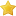•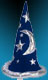• Posts: 13,500
• Joined: 23 Jan 2008
• Loc: Hilton, NY, Yellow Zone (Bortle 4.5)

Posted 05 January 2017 - 03:40 PM

Well, dynamic range is usually thought of in terms of f stops.  For example, the ASI1600 has a maximum dynamic range of about 12.5 stops at zero gain.  You can find this on the chart they provide down this page on their website:

https://astronomy-im...-3-0/asi1600mm/

I'm not sure what the units are for the 2564 number you calculated, or how you would apply it in practice.

-Dan

### #3Andyb90

Andyb90

Vostok 1

••• topic starter
• Posts: 148
• Joined: 21 Aug 2016
• Loc: Staffordshire, UK

Posted 05 January 2017 - 08:13 PM

From what I've read the calculated value is dimensionless. It refers to gray levels/steps. So 4096 would correspond to 12 bit resolution. Presumably 2564 would be 11 bit.

Andy.

### #4Jon Rista

Jon Rista

ISS

••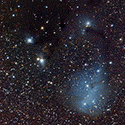• Posts: 24,324
• Joined: 10 Jan 2014

Posted 05 January 2017 - 08:37 PM

Well, dynamic range is usually thought of in terms of f stops.  For example, the ASI1600 has a maximum dynamic range of about 12.5 stops at zero gain.  You can find this on the chart they provide down this page on their website:

https://astronomy-im...-3-0/asi1600mm/

I'm not sure what the units are for the 2564 number you calculated, or how you would apply it in practice.

-Dan

Dynamic range is usually expressed in either decibels or stops. You can calculate the dynamic range in decibels with this formula:

DRdb = 20 * log(FWC/RN)

You can convert that to stops with this formula:

DRstops = DRdb / 6

Dynamic range in "discrete steps" is simply:

DRsteps = FWC/RN

So, if you have an FWC of 4095, and read noise of 1.55e-, your dynamic range would be:

DRdb = 20 * log(4095/1.55) = 20 * log(2641.94) = 20 * 3.422 = 68.44dB

And converting to stops, you would have:

DRstops = 68.44 / 6 = 11.4 stops

For the record, stops and bits, effectively the same thing for all intents and purposes. You can recover bits with stacking, though. You would then divide the read noise by the square root of the number of subs stacked to determine how much bit-recovery potential you have:

BitsStacked = (20 * log(FWC/(RN/SQRT(Subs)))) / 6

So if you have an FWC of 8192, read noise of 2e- and you stack 64 subs:

BitsStacked = (20 * log(8192/(2/SQRT(64)))) / 6 = (20 * log(8192/0.25)) / 6 = (20 * log(32768)) / 6 = (20 * 4.516) / 6 = 90.31 / 6 = 15.05 bits

(NOTE: Technically speaking, dynamic range in stops/bits is also dependent on camera noise from other sources like dark current, glows, etc. And if you want to be truly accurate, the skyfog offset is also going to limit your dynamic range. So the above would be the maximum potential bit depth of a stack if you only had object shot noise and read noise.)

Edited by Jon Rista, 05 January 2017 - 08:44 PM.

• Midnight Dan and ram812 like this

### #5ram812

ram812

Viking 1

••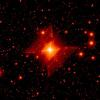• Posts: 786
• Joined: 10 Dec 2014
• Loc: Grants Pass, Oregon

Posted 05 January 2017 - 08:55 PM

Well, dynamic range is usually thought of in terms of f stops.  For example, the ASI1600 has a maximum dynamic range of about 12.5 stops at zero gain.  You can find this on the chart they provide down this page on their website:

https://astronomy-im...-3-0/asi1600mm/

I'm not sure what the units are for the 2564 number you calculated, or how you would apply it in practice.

-Dan

Dynamic range is usually expressed in either decibels or stops. You can calculate the dynamic range in decibels with this formula:

DRdb = 20 * log(FWC/RN)

You can convert that to stops with this formula:

DRstops = DRdb / 6

Dynamic range in "discrete steps" is simply:

DRsteps = FWC/RN

So, if you have an FWC of 4095, and read noise of 1.55e-, your dynamic range would be:

DRdb = 20 * log(4095/1.55) = 20 * log(2641.94) = 20 * 3.422 = 68.44dB

And converting to stops, you would have:

DRstops = 68.44 / 6 = 11.4 stops

For the record, stops and bits, effectively the same thing for all intents and purposes. You can recover bits with stacking, though. You would then divide the read noise by the square root of the number of subs stacked to determine how much bit-recovery potential you have:

BitsStacked = (20 * log(FWC/(RN/SQRT(Subs)))) / 6

So if you have an FWC of 8192, read noise of 2e- and you stack 64 subs:

BitsStacked = (20 * log(8192/(2/SQRT(64)))) / 6 = (20 * log(8192/0.25)) / 6 = (20 * log(32768)) / 6 = (20 * 4.516) / 6 = 90.31 / 6 = 15.05 bits

(NOTE: Technically speaking, dynamic range in stops/bits is also dependent on camera noise from other sources like dark current, glows, etc. And if you want to be truly accurate, the skyfog offset is also going to limit your dynamic range. So the above would be the maximum potential bit depth of a stack if you only had object shot noise and read noise.)

I don't mean to butt-in here as I have a question for Jon.. Are these formulas good for ALL CCD?CMOS/DSLR cameras? Or are there different ones for different types? Also, is there a dedicated astro site with formulas of this nature? Thanks, Ralph

### #6Midnight Dan

Midnight Dan

Voyager 1

••• Posts: 13,500
• Joined: 23 Jan 2008
• Loc: Hilton, NY, Yellow Zone (Bortle 4.5)

Posted 05 January 2017 - 09:06 PM

Well, dynamic range is usually thought of in terms of f stops.  For example, the ASI1600 has a maximum dynamic range of about 12.5 stops at zero gain.  You can find this on the chart they provide down this page on their website:

https://astronomy-im...-3-0/asi1600mm/

I'm not sure what the units are for the 2564 number you calculated, or how you would apply it in practice.

-Dan

Dynamic range is usually expressed in either decibels or stops. You can calculate the dynamic range in decibels with this formula:

DRdb = 20 * log(FWC/RN)

You can convert that to stops with this formula:

DRstops = DRdb / 6

Dynamic range in "discrete steps" is simply:

DRsteps = FWC/RN

So, if you have an FWC of 4095, and read noise of 1.55e-, your dynamic range would be:

DRdb = 20 * log(4095/1.55) = 20 * log(2641.94) = 20 * 3.422 = 68.44dB

And converting to stops, you would have:

DRstops = 68.44 / 6 = 11.4 stops

For the record, stops and bits, effectively the same thing for all intents and purposes. You can recover bits with stacking, though. You would then divide the read noise by the square root of the number of subs stacked to determine how much bit-recovery potential you have:

BitsStacked = (20 * log(FWC/(RN/SQRT(Subs)))) / 6

So if you have an FWC of 8192, read noise of 2e- and you stack 64 subs:

BitsStacked = (20 * log(8192/(2/SQRT(64)))) / 6 = (20 * log(8192/0.25)) / 6 = (20 * log(32768)) / 6 = (20 * 4.516) / 6 = 90.31 / 6 = 15.05 bits

(NOTE: Technically speaking, dynamic range in stops/bits is also dependent on camera noise from other sources like dark current, glows, etc. And if you want to be truly accurate, the skyfog offset is also going to limit your dynamic range. So the above would be the maximum potential bit depth of a stack if you only had object shot noise and read noise.)

OK Jon ... when are you going to yield to your true calling and teach a college course on this stuff?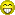-Dan

### #7Jon Rista

Jon Rista

ISS

••• Posts: 24,324
• Joined: 10 Jan 2014

Posted 05 January 2017 - 09:13 PM

They are good for general approximation for any camera, assuming you know the specifics of the sensor's behavior. The original formula, FWC/RN, will give you the CAMERA dynamic range. That is a hardware trait.

The formula for the number of bits in a stack of frames is, as I mentioned, really dependent on more factors. Dark current at the very least, and really skyfog as well, also reduce dynamic range, and also make it harder to recover bit depth (since you are starting with even fewer effective bits than the base dynamic range of the camera.)

### #8Andyb90

Andyb90

Vostok 1

••• topic starter
• Posts: 148
• Joined: 21 Aug 2016
• Loc: Staffordshire, UK

Posted 06 January 2017 - 06:31 AM

I found an interesting webpage here:

https://www.microsco...digital-imaging

Here is a quote from one section of the page:

"In a 12-bit digital camera, the analog signal from the CCD is digitized with 12-bit depth by the on-board A/D converter. Whether or not the output can actually be resolved into 4096 discrete intensity levels (12 bits) depends on the camera noise. In order to discriminate between individual intensity levels, each gray level step should be about 2.7 times larger than the camera noise. Otherwise, the difference between steps 2982 and 2983, for example, cannot be resolved with any degree of certainty. Some so-called 12-bit cameras have so much camera noise that 4096 discrete steps cannot be discriminated"

So if that is correct higher read noise can prevent resolution of the individual steps/levels?

Andy.

Edited by Andyb90, 06 January 2017 - 06:32 AM.

### #9Jon Rista

Jon Rista

ISS

••• Posts: 24,324
• Joined: 10 Jan 2014

Posted 06 January 2017 - 11:18 AM

I found an interesting webpage here:

https://www.microsco...digital-imaging

Here is a quote from one section of the page:

"In a 12-bit digital camera, the analog signal from the CCD is digitized with 12-bit depth by the on-board A/D converter. Whether or not the output can actually be resolved into 4096 discrete intensity levels (12 bits) depends on the camera noise. In order to discriminate between individual intensity levels, each gray level step should be about 2.7 times larger than the camera noise. Otherwise, the difference between steps 2982 and 2983, for example, cannot be resolved with any degree of certainty. Some so-called 12-bit cameras have so much camera noise that 4096 discrete steps cannot be discriminated"

So if that is correct higher read noise can prevent resolution of the individual steps/levels?

Andy.

It is actually better to outresolve the noise if you can. This is why 16-bit ADC units are usually used with CCD cameras despite the fact that most have 11-12 stops of dynamic range. Ideally, you would want a gain of 0.5e-/ADU, which would mean each individual electron produced 2 ADU. Then, due to noise and quantization error, the noise itself would be finely sampled around 1 ADU.

In the case of the ASI1600, it has 3.5e- read noise and 20,000e- FWC at Gain 0. The quantization error is 4.88e-/ADU. This means the camera radically undersamples each electron, and even undersamples the noise, so you cannot fully achieve the 12.52 stops of dynamic range the camera is capable of. A large quantization error limits you to 12 stops of dynamic range. If on the other hand the camera had a 14- or 16-bit ADC, the full dynamic range would have been realized.

## CNers have asked about a donation box for Cloudy Nights over the years, so here you go. Donation is not required by any means, so please enjoy your stay.## Recent TopicsCloudy Nights LLC Cloudy Nights Sponsor: Astronomics